# IB DP Physics: SL复习笔记8.1.5 Energy Generation

### Energy Generation

• You need to know about the main ways of generating electricity:
• The equipment involved
• Calculate power obtained for different setups
• These revision notes cover:
• Nuclear Power
• Burning Fossil Fuels
• Wind Electricity Generators
• Hydroelectric Power
• Solar Power

#### Nuclear Power

Control Rods & Moderators:

• In a nuclear reactor, a chain reaction is required to keep the reactor running
• When the reactor is producing energy at the correct rate, two factors must be controlled:
• The number of free neutrons in the reactor
• The energy of the free neutrons
• To do this, nuclear reactors contain control rods and moderators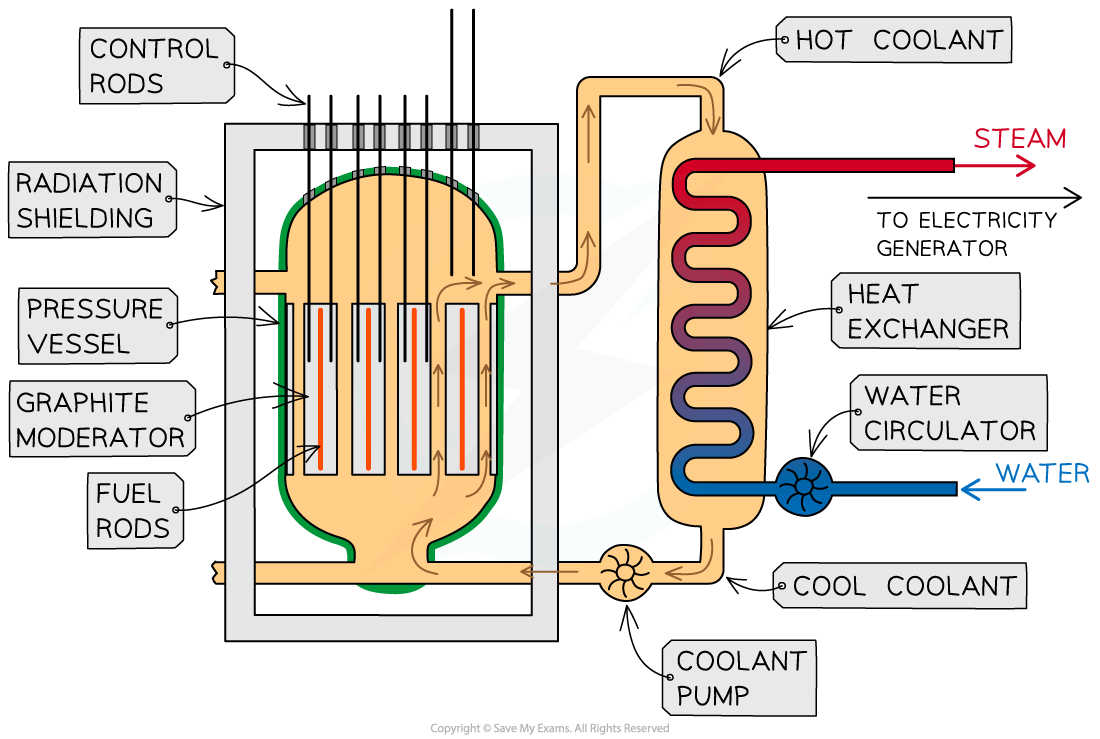The overall purpose of a nuclear reactor is to collect the heat energy produced from nuclear reactions

Control Rods:

Purpose of a control rod: To absorb neutrons

• Control rods are made of a material which absorb neutrons without becoming dangerously unstable themselves
• The number of neutrons absorbed is controlled by varying the depth of the control rods in the fuel rods
• Lowering the rods further decreases the rate of fission, as more neutrons are absorbed
• Raising the rods increases the rate of fission, as fewer neutrons are absorbed
• This is adjusted automatically so that exactly one fission neutron produced by each fission event goes on to cause another fission
• In the event the nuclear reactor needs to shut down, the control rods can be lowered all the way so no reaction can take place

Moderator:

The purpose of a moderator: To slow down neutrons

• The moderator is a material that surrounds the fuel rods and control rods inside the reactor core
• The fast-moving neutrons produced by the fission reactions slow down by colliding with the molecules of the moderator, causing them to lose some momentum
• The neutrons are slowed down so that they are in thermal equilibrium with the moderator, hence the term ‘thermal neutron’
• This ensures neutrons can react efficiently with the uranium fuel

Shielding:

• The entire nuclear reactor is surrounded by shielding materials
• The purpose of shielding is to absorb hazardous radiation
• The daughter nuclei formed during fission, and the neutrons emitted, are radioactive
• The reactor is surrounded by a steel and concrete wall that can be nearly 2 metres thick
• This absorbs the emissions from the reactions
• It ensures that the environment around the reactor is safe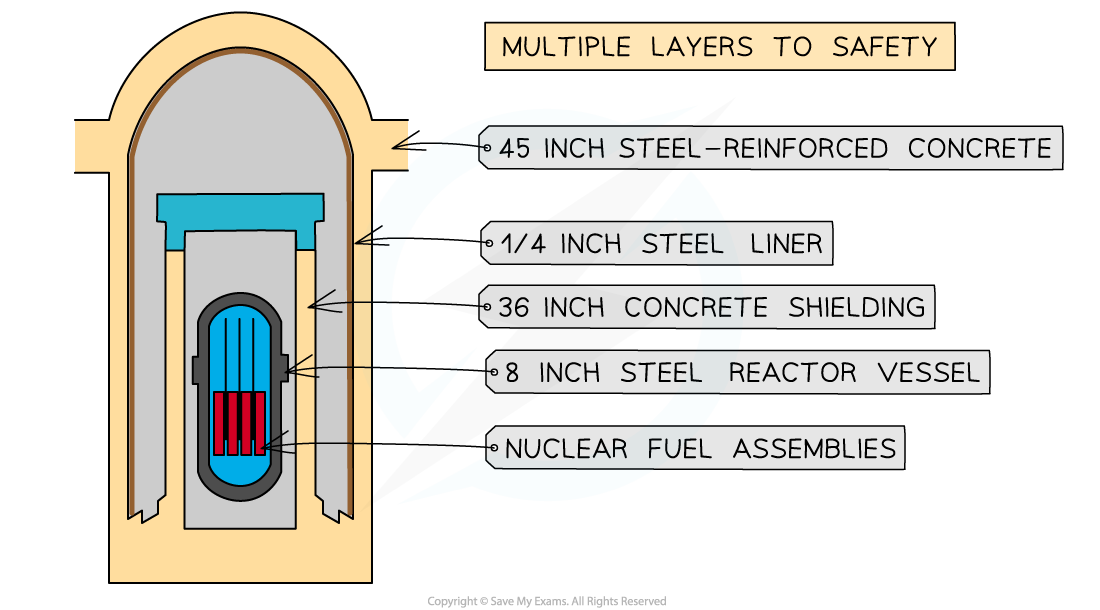Shielding metals in a nuclear reactor

#### Advantages & Disadvantages of Nuclear Power

• Advantages of using nuclear power include:
• Extensive reserves of fissionable materials
• Increasingly refine technology available
• No greenhouse gases produced
• A large amount of power is produced
• Disadvantages of using nuclear power include:
• Hazardous radioactive waste materials produced
• Dangerous if the power plant goes significantly wrong
• Danger of misuse of nuclear material
• Problems with mining fuel

#### Burning Fossil Fuels

• Fossil fuels, such as coal and oil, are used to produce energy on-demand when energy is needed
• This is done by burning the materials when the energy is required
• When fossil fuels are burned, it is used to heat water
• This water is heated until it becomes steam
• Steam is forced around the system and this turns a turbine
• The turbine spins and is connected to a generator which generates electricity
• This electricity is carried out of the system by electrical lines
• The steam within the turbine will cool and condense and then be pumped back into the boiler to repeat the process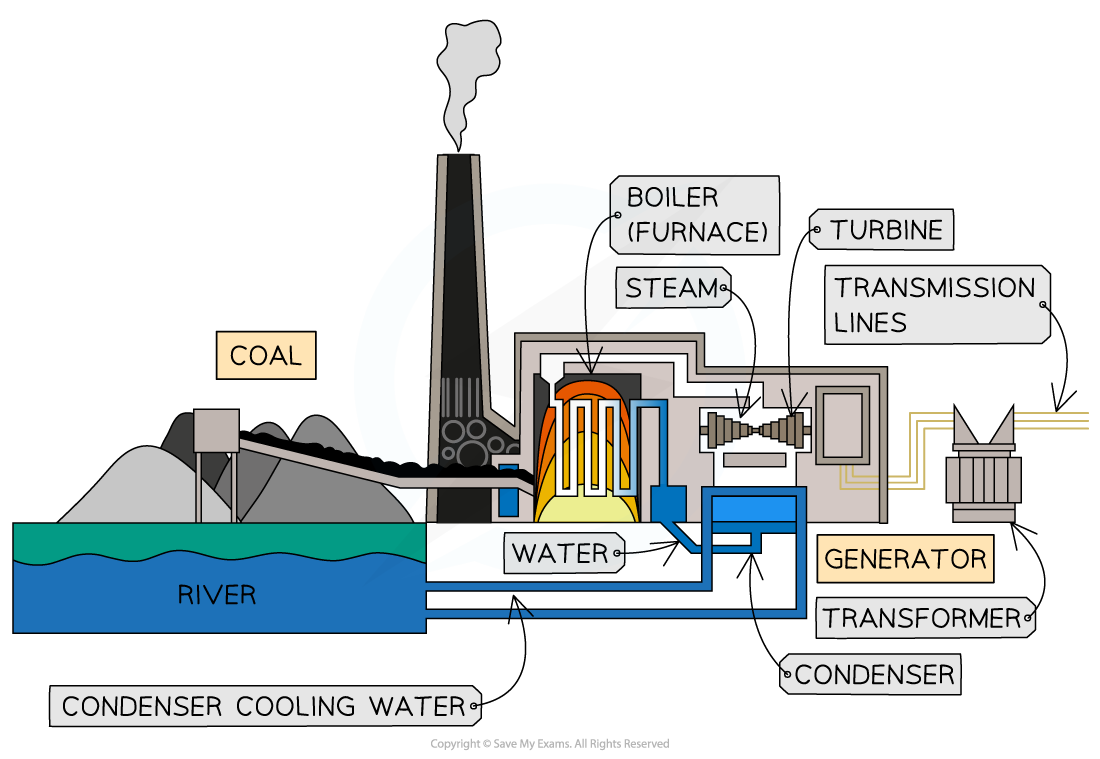Diagram of a Fossil fuel based Reactor. The overall purpose of the reactor is to collect the heat energy produced from burning fossil fuels

• The approximate efficiency of fossil-fuel based power plants is approximately 40%
• Energy is lost to heat within exhaust gases, heat loss in the condenser process and friction within the system

#### Advantages & Disadvantages of Fossil Fuels

• Advantages of using fossil fuel based power plants include:
• Extensive infrastructure in place
• High energy density of fuel
• Available energy at any time
• Well-known and developed technology
• Disadvantages of using fossil fuel based power plants include:
• Produces greenhouse gases
• Unsustainable (non-renewable)
• Produces pollution

#### Wind Electricity Generators

• Wind generators can be principally horizontally or vertically aligned
• The majority of modern designs use horizontally aligned designs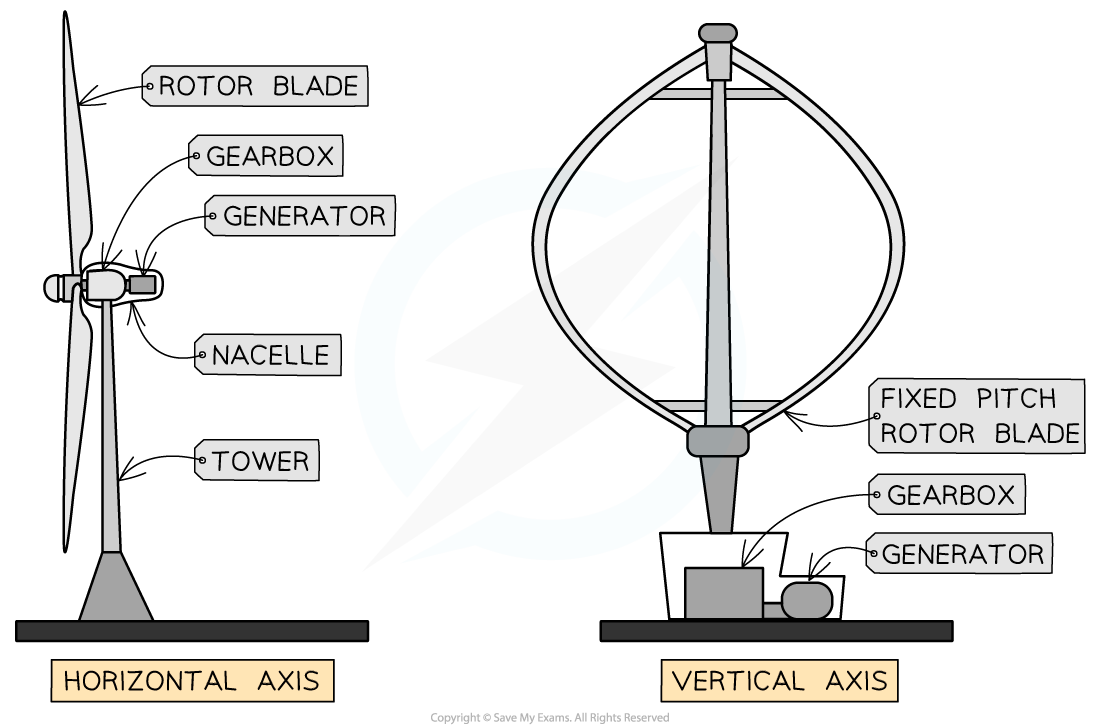The two main designs of wind generators: horizontal and vertical alignment

• The approximate efficiency of wind generators is approximately 30%
• Energy is lost to aerodynamic limits, losses transferring the electricity to the grid and friction within the system

#### Advantages & Disadvantages of Wind Power

• Advantages of using wind-powered generators include:
• Clean (non-polluting) energy generation
• Freely available
• Is always sustainable and will never run out
• Disadvantages of using wind-powered generators include:
• Not consistent energy production
• Needs favourable local conditions to be placed in windy locations
• Can be visually unappealing

#### Hydroelectric Power

• Hydroelectric power using water stored at a height h, that mass of water m, is allowed to flow through turbines being pulled down by the acceleration due to gravity g
• The falling water has stored gravitational potential energy which is released when falling and used to spin turbines that generate electricity
• Energy can be stored for later use by pumping water back up to a higher location to be released to a lower location and spinning the turbines again when needed
• The approximate efficiency of hydroelectric power generation is approximately 90%
• Energy is lost to friction and other resistive forces
• The approximate maximum power that can be generated from a hydroelectric generator can be estimated by considering the rate of change of potential energy of the water falling through the turbines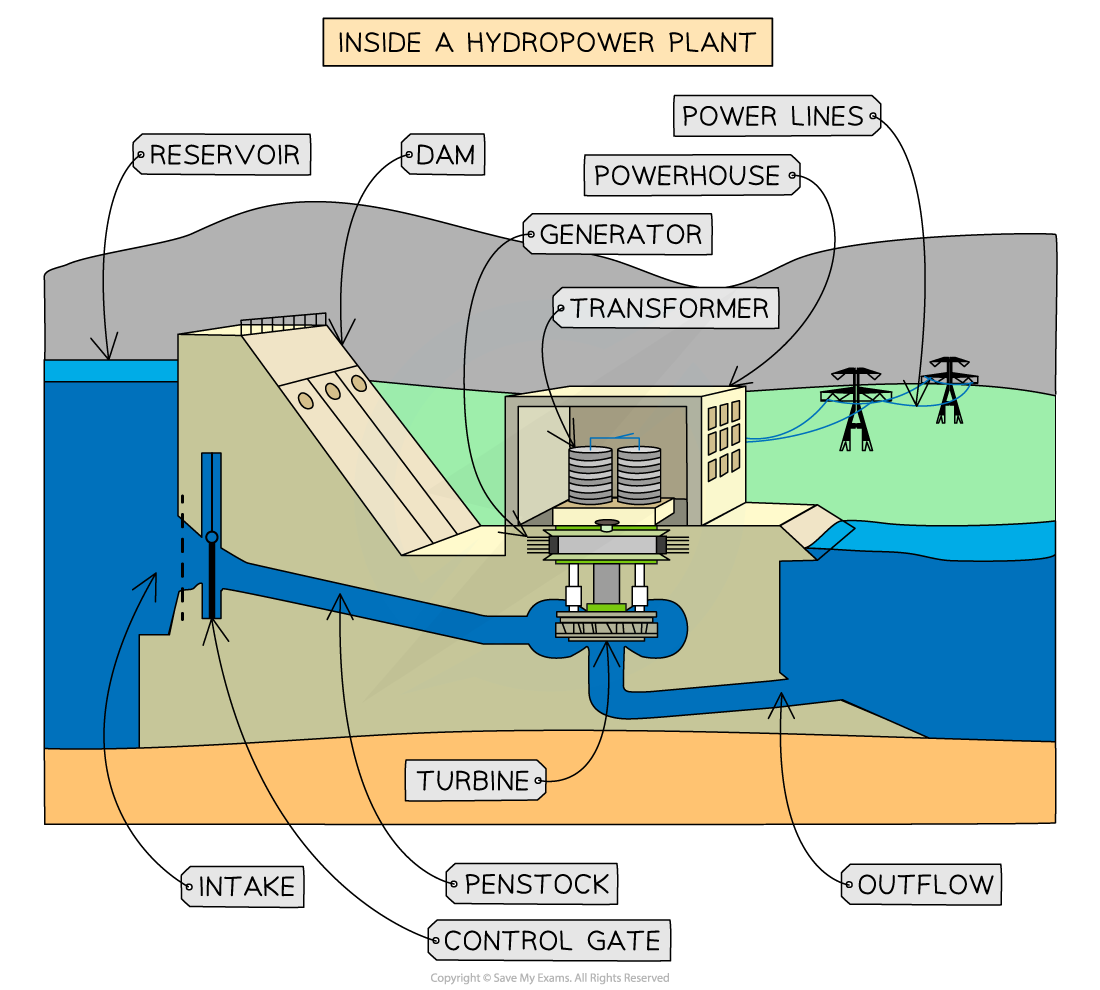A hydroelectric power generation station. In off-peak hours water can be pumped back to the higher reservoir.

#### Advantages & Disadvantages of Hydroelectric Power

• Advantages of using hydroelectric generators include:
• Clean (non-polluting) energy generation
• Is sustainable
• Can be stored for when needed
• Disadvantages of using hydroelectric generators include:
• Large areas and changes to the environment are needed
• It relies on suitable locations
• A large initial investment is required

### Calculating Energy Transformations

#### Wind Electricity Generators

• The approximate maximum power obtained per second that can be generated from a horizontal wind generator can be estimated by considering the blade radius and an incoming column of airA column of air can provide only a limited amount of energy for a wind generator of blade radius r

• A column of air of density ρ can move through a cross-sectional area A which is determined by the blade radius r
• The amount of air that can move through this region is dependent on the velocity of the air v and the time considered t
• The kinetic energy of the air arriving at the turbine every second can be described by:

KE = ½ × mass × velocity2

• The mass, m, of the column of air can be described by using the equation

m = ρV = ρAL

• Where:
• ρ = density of the air (kg m−3)
• V = volume of the column (m3)
• A = cross-sectional area of the column (m2)
• L = length of the column (m)
• The length of the column can be described by

L = vt

• Where:
• v = velocity of the air (m s−1)
• t = time taken to travel the length of the column (s)
• Since the case is being considered for every second, time, t = 1 s
• Therefore, mass, in this case, will be:

m = ρvtA = ρvA

• This means power obtained per second can be described by:

P = ½ × (ρvA) × v2

P = ½ ρAv3

• This equation shows that the main variable that can impact power generated by wind is the wind's velocity
• If the wind's velocity remains the same, but the area covered by the blades doubles, then the theoretical power will double
• Yet, if the area covered by the blades remains the same and the wind velocity doubles, then the theoretical power available will increase eight-fold
• Typical values of quantities are useful to be aware of:
• Density of the air = 1.3 kgm−3 at standard temperature and pressure
• Velocity of the air required to turn the blades = 12 kmh−1 to get them turning and then 70 kmh−1 at full capacity
• Radius of the wind turbine blade = 50 - 150 m

#### Worked Example

Air moving at speed 9.5 m s-1 with a density of 1.15 kg m-3 is incident on a wind turbine. The length of the blades in the wind turbine are 14 m. After passing the wind turbine, the air is moving with a speed of 4.5 m s-1 and has a density of 1.30 kg m-3. Deduce the maximum power possible every second from this situation.

Step 1: List known values

• Air velocity before passing turbine: 9.5 m s-1
• Air density: 1.15 kg m-3
• Blade length: 14 m
• Air velocity after passing turbine: 4.5 m s-1
• Density: 1.30 kg m-3

Step 2: Find maximum power available

P = 0.5 × ρ × A × v3 = 0.5 × 1.15 × π × 142 × 9.53 = 3.04 × 105W

Step 3: Find the remaining power within the air after passing the turbine

P = 0.5 × ρ × A × v3= 0.5 × 1.15 × π × 142 × 4.53= 3.64 × 104 W

Step 4: Find the power available for the turbine to use

P = (3.04 × 105) - (3.64 × 104) = 2.68 × 105 W

Step 5: State the final answer

• The maximum power available to the turbine is: 2.68 × 105 W every second

#### Hydroelectric Power

• Consider water of mass m stored at a height h, that is allowed to flow through turbines being pulled down by the acceleration due to gravity g
• The gravitational potential energy of the water is m × g × h
• Therefore, the change in energy (the power) will be:• Where Δt = the time taken for the change in energy to occur
• This can be re-written in terms of volume and density rather than mass to consider the equation in terms of the volume flow rate• Substituting this into the power equation gives:• Therefore, to get large energy from hydroelectric power, large flow rates (ΔV ÷ Δt) and heights h are needed to maximise the energy produced

#### Worked Example

In a hydroelectric dam, water of density 1000 kg m−3flows with a flow rate: 75 × 10−3 m3 s−1goes through a turbine and descends approximately 22 m. Deduce the maximum power this will give if the efficiency of the turbine system is 85%.

Step 1: List the known quantities

• Flow rate, ΔV / Δt = 75 × 10−3 m3 s−1
• Height of water drop, h = 22 m
• Density of water, ρ = 1000 kg m−3
• Efficiency of turbine system, e = 85%

Step 2: State the relevant equation for hydroelectric power• P = power (W)
• ρ = density (kg m-3)
• g = acceleration due to gravity (m s−2)
• h = height of falling water (m)
• ΔV / Δt = the flow rate (m3 s−1)
• e = efficiency [no units]

Step 3: Substitute in the values

P = (1000 × 9.8 × 22) × (75 × 10−3) × 0.85 = 1.37 x 104 W

Step 4: State final answer

• The approximate power available for the situation is: 1.37 × 104 W